2015 September 12
Fun fact: the sum of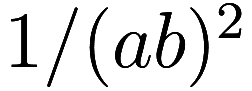over all relatively prime pairs of positive integers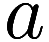,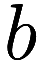is rational. This is not true if you remove the “relatively prime” restriction.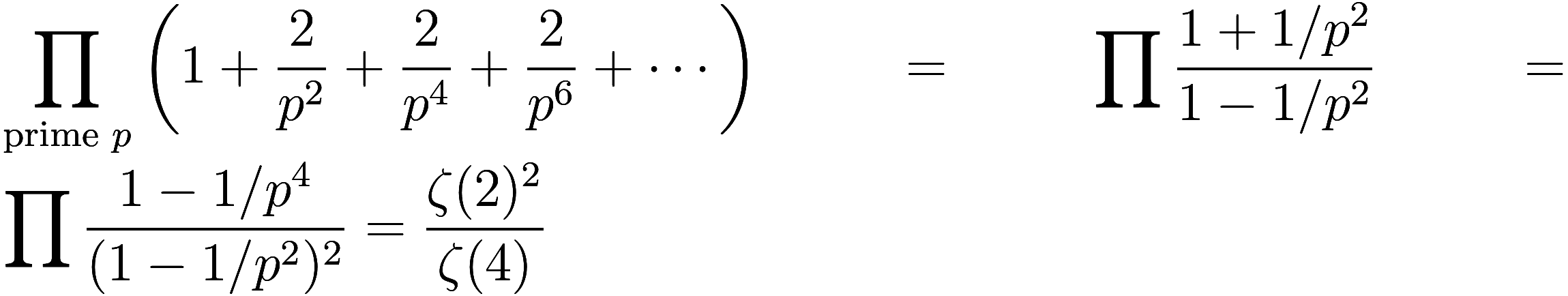where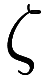is the Riemann zeta function. Since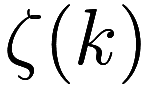is equal to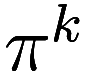times a rational when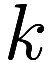is positive even, the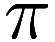s cancel and the result is rational, in fact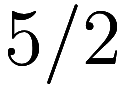. If you remove the restriction you get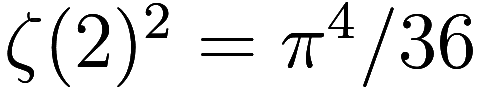which is irrational. You can make other combinations ofs so that thes cancel to get other rational infinite summations, for example the sum of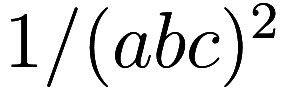whereis squarefree and dividesshould give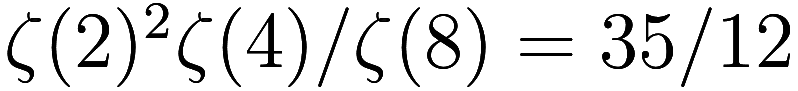, and in both examples replacing the exponent 2 by any even integer also works.